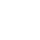# “千古词帝”李煜诗词全集

“千古词帝”李煜诗词全集

[] 李煜[] 李煜

[] 李煜

[] 李煜

[] 李煜

[] 李煜[] 李煜

[] 李煜

[] 李煜

[] 李煜

[] 李煜

[] 李煜

（一名一箩金、黄金缕、明月生南浦、凤栖梧、

[] 李煜

[] 李煜

[] 李煜[] 李煜

[] 李煜

[] 李煜

[] 李煜

[] 李煜

[] 李煜

[] 李煜

[] 李煜

[] 李煜[] 李煜

[] 李煜

[] 李煜

[] 李煜[] 李煜

（一名望江南、梦江南、江南好、梦江口、望江梅、

[] 李煜

[] 李煜

[] 李煜

[] 李煜[] 李煜

[] 李煜

[] 李煜

[] 李煜

[] 李煜

[] 李煜

[] 李煜

[] 李煜

[] 李煜

[] 李煜[] 李煜

[] 李煜

[] 李煜

[] 李煜

[] 李煜

[] 李煜

[] 李煜[] 李煜

[] 李煜

[] 李煜

[] 李煜

[] 李煜

[] 李煜

[] 李煜

[] 李煜

[] 李煜

[] 李煜

[] 李煜

[] 李煜

[] 李煜

[] 李煜

[] 李煜

[] 李煜“千古词帝”李煜诗词全集
“千古词帝”李煜诗词全集长按图片保存/分享
0电话：0851-33339999  18808530000（微信）  邮箱：18985300000@qq.com• 取消“扫一扫”加入群聊